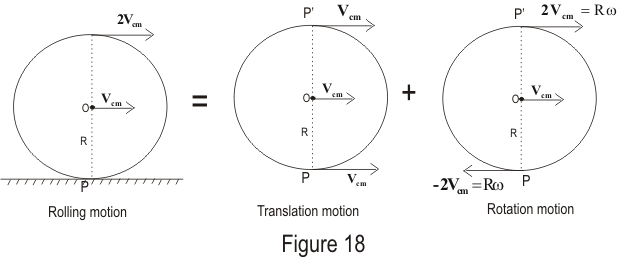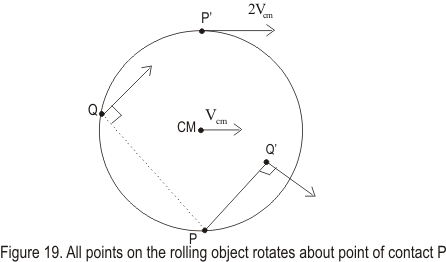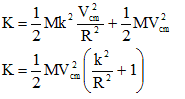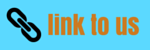# Rotation

## (14) Radius of gyration

• Whatever may be the shape of the body it is always possible to find a distance from the axis of rotation at which whole mass of the body can be assumed to be concentrated and even then its moment of inertia about that axis remains unchanged.
• If whole mass of the body is supposed to be concentrated at a distance k from the axis of rotation then
I=Mk2=Σmr2
or ,
k=√(I/M)=√(Σmr2/M)
This quantity k is called radius of gyration of the body about the axis of rotation.
• Thus, the radius of gyration of a body, rotating about a given axis of rotation is the radial distance from the axis and when the square of radius of gyration (k) is multiplied by the total mass of the body it gives the moment of inertia of the body about that axis.

## (15) Kinetic Energy of rolling bodies (rotation and translation combined)

• Let us now calculate the kinetic energy of a rolling body. For this consider a body with circular symmetry for example cylinder, wheel, disc , sphere etc.
• When such a body rolls on a plane surface , the motion of such a body is a combination of translational motion and rotational motion as shown below in the figure.• At any instant the axis normal to the digram through the point of contact P is the axis of rotation. If the speed of the centre of mass relative to an observer fixed on the surface is Vcm then the instantaneous angular speed about an axis through P would be
ω=Vcm/R
where R is the radius of the body.
• To explain this consider the figure given belowAt any instant different particles of the body have different linear speeds. The point P is at rest Vcm=0 instantaneously , the centre of mass has speed Vcm=Rω and the highest point on the circumfrance p' has speed Vcm=2Rω relative to point P.
• Now again consider the first figure the top of the cylinder has linear speed Vcm+ Rω=Vcm+Vcm=2Vcm, which is greater than the linear speed of any other point on the cylinder. We thus note that the center of mass moves with linear speed Vcm while the contact point between the surface and rolling objectr has a linear speed of zero.
• Therefore at that instant all particles of the rigid body are moving with the same angular speed ω about the axis through P and the motion of the body is equivalent to pure rotational motion.
• Thus total kinetic energy is
K=½(IPω2)
where IP is the moment of inertia of the rigid body about point P.
• From parallel axis theorem
IP=Icm+MR2
where Icm is the moment of inertia of the body of mass M about parallel axis through point O.
Therefore
K=½(Icmω2)+½(MR2ω2)=½(Icmω2)+½(M(Vcm)2)
here the first term represents the rotational kinetic energy of the cylinder about its center of mass, and the second term represents the kinetic energy the cylinder would have if it were just translating through space without rotating. Thus, we can say that the total kinetic energy of a rolling object is the sum of the rotational kinetic energy about the center of mass and the translational kinetic energy of the center of mass.
• If k is the radius of gyration of the body about a parallel axis through O then I=Mk2 and total kinetic energy would then be,